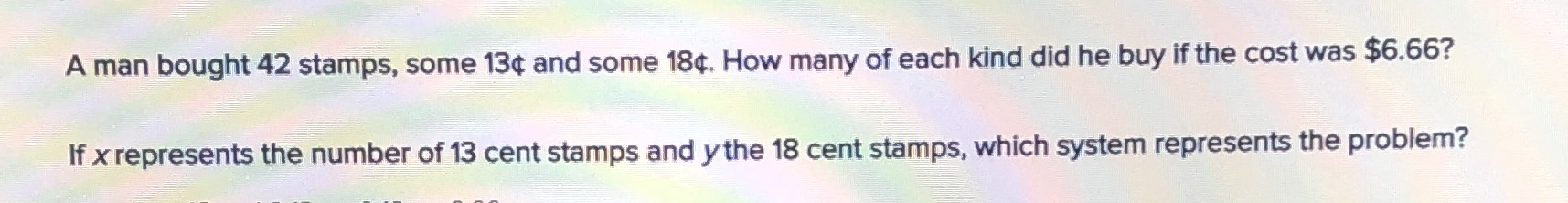### ¿Todavía tienes preguntas de matemáticas?

Pregunte a nuestros tutores expertos
Algebra
PreguntaA man bought $$42$$ stamps, some $$13 \%$$ and some $$18 \%$$ . How many of each kind did he buy if the cost was $$\ 6.66 ?$$ If $$x$$ represents the number of $$13$$ cent stamps and $$y$$ the $$18$$ cent stamps, which system represents the problem?